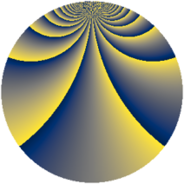# Properties

 Label 2678.2.boLevel $2678$ Weight $2$ Character orbit 2678.bo Rep. character $\chi_{2678}(25,\cdot)$ Character field $\Q(\zeta_{102})$ Dimension $3840$ Sturm bound $728$

# Related objects

## Defining parameters

 Level: $$N$$ $$=$$ $$2678 = 2 \cdot 13 \cdot 103$$ Weight: $$k$$ $$=$$ $$2$$ Character orbit: $$[\chi]$$ $$=$$ 2678.bo (of order $$102$$ and degree $$32$$) Character conductor: $$\operatorname{cond}(\chi)$$ $$=$$ $$1339$$ Character field: $$\Q(\zeta_{102})$$ Sturm bound: $$728$$

## Dimensions

The following table gives the dimensions of various subspaces of $$M_{2}(2678, [\chi])$$.

Total New Old
Modular forms 11776 3840 7936
Cusp forms 11520 3840 7680
Eisenstein series 256 0 256

## Trace form

 $$3840q - 120q^{4} - 200q^{9} + O(q^{10})$$ $$3840q - 120q^{4} - 200q^{9} - 8q^{10} - 4q^{13} - 8q^{14} + 120q^{16} - 4q^{17} + 8q^{22} - 120q^{25} + 6q^{26} - 8q^{29} - 12q^{35} - 236q^{36} + 4q^{38} - 76q^{39} - 4q^{40} + 16q^{42} - 16q^{43} - 96q^{49} - 2q^{52} - 20q^{53} + 36q^{55} - 4q^{56} - 48q^{61} + 28q^{62} + 240q^{64} - 8q^{65} - 56q^{66} + 4q^{68} + 152q^{69} - 8q^{74} + 8q^{75} - 212q^{77} - 2q^{78} + 48q^{79} - 96q^{81} + 4q^{82} + 36q^{87} + 4q^{88} - 96q^{90} - 222q^{91} + 160q^{94} - 40q^{95} + O(q^{100})$$

## Decomposition of $$S_{2}^{\mathrm{new}}(2678, [\chi])$$ into newform subspaces

The newforms in this space have not yet been added to the LMFDB.

## Decomposition of $$S_{2}^{\mathrm{old}}(2678, [\chi])$$ into lower level spaces

$$S_{2}^{\mathrm{old}}(2678, [\chi]) \cong$$ $$S_{2}^{\mathrm{new}}(1339, [\chi])$$$$^{\oplus 2}$$